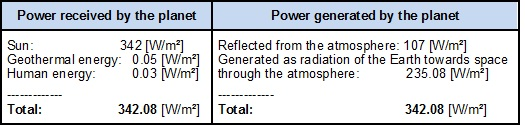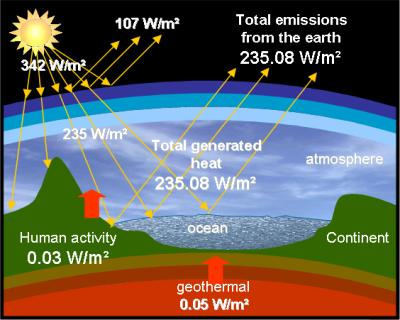Chargement...

# 5) The thermal imbalance 5) The thermal imbalance

5) The thermal imbalance

The fact that the temperature of our planet is increasing signals a thermal imbalance, because if the amounts of energy received and energy lost were equal then its temperature would remain constant.

What happens when the equilibrium between the heat received and the heat lost by a system is broken?If a system receives more heat than it emits, its temperature increases until its losses equal its gains.

Therefore if the planet receives more energy (from outside or from inside) than it emits, its temperature can only increase, which leads to an increase of its losses, until a new equilibrium is found at a higher temperature.

Therefore if we stabilize the amount of heat generated by human activity to its current value of 1.5 х E13 [W] (i.e. 0.03 [m²] ), the temperature of the Earth will rise until this increase leads to an equal additional emission of heat towards space. We will then reach the following balance: